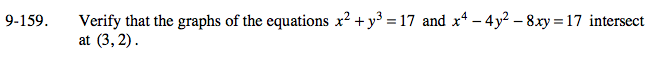### Home > A2C > Chapter 9 > Lesson 9.3.2 > Problem9-159

9-159.Insert the values of the point into the equations.

32 + 23 = 17
34 − 4(22) − 8(3)(2) = 17

Simplify.

9 + 8 = 17

81 − 16 − 48 = 17

17 = 17
17 = 17